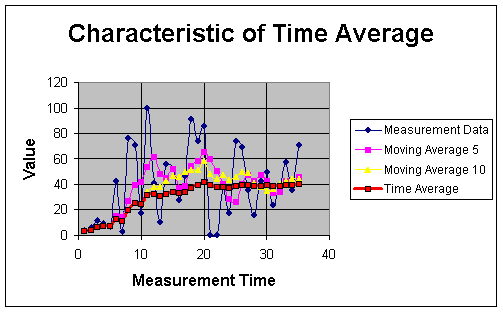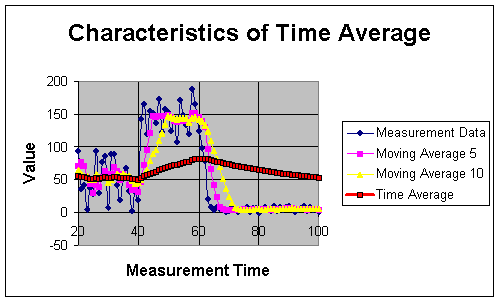## Characteristics of Time Average

To show the characteristics of time-average, we create random measurement data and plot the data together with the moving average 5 and 10 consecutive data and the time average.In the graph above, the measurement data was fluctuated randomly from zero to 100. Higher number of data to be averaged make the moving average goes smoother. Time average, however, is the smoothest moving average. It goes directly to the core of the data and lazy to move away from there. As the data fluctuated very much, the moving average will follow the data fluctuation. The time average, however, do not easily deviate away by the fluctuation of the data.In above graph, we change the measurement data. From measurement time 20 to 40, the data is random between 0 to 100; from measurement time 40 to 60, the data value is randomly fluctuated between 100 to 200 and above 60, the data is random between 0 to 10. As usual the moving average will smooth the data while following the fluctuation of the measurement data. The time average, however, is about the average of the data (around 50) between measurement time 20 to 40. When the measurement move away suddenly above 100, the time average follow to increase with a very slow performance due to long history. Unfortunately, before the time average can reach the average of the data, the measurement data has change again to a very small (below 10). The time average again follow to decrease with a very slow change. Thus, the time average has a long term memory.

Preferable reference for this tutorial is

Teknomo, Kardi. (2006) Recursive Average and Variance.
http://people.revoledu.com/kardi/tutorial/RecursiveStatistic/index.html